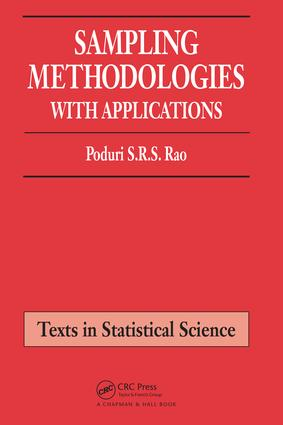# Sampling Methodologies with Applications

## 1st Edition

Chapman and Hall/CRC

336 pages | 20 B/W Illus.

##### Purchasing Options:\$ = USD
Paperback: 9781584882145
pub: 2000-11-22
\$140.00
x
Hardback: 9781138462557
pub: 2017-08-09
\$205.00
x
eBook (VitalSource) : 9781466594517
pub: 2013-01-29
from \$67.50

FREE Standard Shipping!

### Description

Sampling methods are integral to the design of surveys and experiments, to the validity of results, and thus to the study of statistics, social science, and a variety other disciplines that use statistical data. Yet most of the available texts on the subject are either quite advanced and theoretical or too applied, descriptive, and lacking statistical results.

Sampling Methodologies with Applications offers a balanced, practical treatment of the techniques and applications of the commonly used procedures for sampling from finite populations. It keeps mathematics to a minimum, but does not avoid them entirely: it features the principle results within the text but provides their derivations in the Appendices to each chapter. In an easily followed, step-by-step presentation, the author motivates each topic with illustrations followed by examples and exercises. All of these are constructed from everyday, practical situations covering a wide variety of topics, from scholastic aptitude tests to healthcare expenditures and presidential elections.

Why wade through advanced, theoretical tomes when what you need is straightforward, practical information? Why risk missing important statistical results often omitted from more basic texts? Sampling Methodologies with Applications has everything you need, presented clearly and logically for quick access to topics central to actual practice.

INTRODUCTION

Censuses and Surveys

Types of Surveys

Sampling Frame

Questionnaires, Interviews, and Sample Sizes

Probability Sampling

Nonprobability Sampling

Sampling in Practice

SIMPLE RANDOM SAMPLING: ESTIMATION OF MEANS AND TOTALS

Population Total, Mean and Variance

Sampling without Replacement

Sample Mean and Variance

Properties of Simple Random Sampling

Unbiasedness of the Sample Mean and Variance

Standard Error of the Sample Mean

Distribution of the Sample Mean

Confidence Limits for the Mean

Estimating the Total

Coefficient of Variation of the Sample Mean

Sample Size Determination

Sample Sizes for Individual Groups

SIMPLE RANDOM SAMPLING: RELATED TOPICS

Bias, Variance and Mean Square Error (MSE) of an Estimator

Precision, Accuracy and Consistency

Covariance and Correlation

Linear Combination of Two Characteristics

Systematic Sampling

Nonresponse

Inference Regarding the Means

Sampling with Replacement

PROPORTIONS, PERCENTAGES, AND COUNTS

Two Classes

Total Number or Count

Confidence Limits

Sample Sizes for Specified Requirements

Political and Public Polls

Estimation for more than Two Classes

Combining Estimates from Surveys

Covariances of Proportions

Difference between Proportions

Sample Sizes for more than Two Classes

Estimating Population Size

STRATIFICATION

Notation

Estimation for a Single Stratum

Estimation of the Population Mean and Total

Confidence Limits

Proportions and Totals

Population Total and Proportion

Proportional and Equal Allocation of the Sample

Neyman Allocation

Gains from Neyman Allocation

Summary on the Precisions of the Allocations

Sample Size Allocation to Estimate Proportions

Sample Size to Estimate Means and Totals

Sample Sizes to Estimate Proportions

Sample Sizes for Minimizing Variance or Cost

Further Topics

SUBPOPULATIONS

Totals, Means, and Variances

Estimation of the Means and their Differences

Totals of Subpopulations

Sample Sizes for Estimating the Means and Totals

Proportions and Counts

Subpopulations of a Stratum

Totals and Means of Subpopulations in Strata

Stratification: Proportions and Counts of Subpopulations

CLUSTER SAMPLING

Clusters of Equal Sizes

Estimation of the Means

Comparison with Simple Random Sampling

Estimation of the Standard Error

Optimum Cluster and Sample Sizes

Unequal Size Clusters

Alternative Estimation with Unequal Sizes

Proportions and Percentages

Stratification

Unequal Probability Selection

Horvitz-Thompson Estimator

Alternative Approaches

SAMPLING IN TWO STAGES

Equal Size First Stage Units

Estimating the Mean

Sample Size Determination - Equal size PSUs

Unequal Size Primary Units

Sample Sizes - Unequal Size PSUs

Ratio Adjustment for the Sizes

Proportions and Totals

Unequal Probability Selection

RATIOS AND RATIO ESTIMATORS

Bias and Variance of the Sample Ratio

Confidence Limits for the Ratio of Means

Ratio Estimators for the Mean and Total

Confidence Limits for the Mean and Total

Differences between Ratios, Means, or Totals

Regression through the Origin and the BLUEs

Ratio Estimation versus Stratification

Ratio Estimation with Stratification

Bias Reduction

Two-phase or Double Sampling Ratio Estimators

Ratio Estimator with Unequal Probability Selection

Multivariate Ratio Estimator

REGRESSION ESTIMATION

The Regression Estimator

Estimation from the Sample

Classical Linear Regression

Differences between Regression Estimators

Regression Estimation versus Stratification

Stratification and Regression Estimator

Multiple Regression Estimator

Double Sampling Regression Estimator

Generalized Regression and Calibration Estimators

NONRESPONSE AND REMEDIES

Effects of Survey Topics and Interviewing Methods

Response Rates

Bias and MSE

Estimating Proportions

Subsampling the Nonrespondents

Estimating the Missing Observations

Ratio and Regression Estimators

Poststratification and Weighting

Response Probabilities and Weighting

Imputation

Related Topics

FURTHER TOPICS

Linearization

The Jackknife

The Bootstrap

Balanced Repeated Replication (BRR)

Small Area Estimation

Complex Surveys

SOLUTIONS TO EXERCISES

BIBLIOGRAPHY

APPENDIX OF TABLES

NOTE: Each chapter also contains an Introduction, Exercises and Appendix

### Subject Categories

##### BISAC Subject Codes/Headings:
MAT029000
MATHEMATICS / Probability & Statistics / General